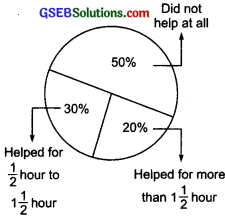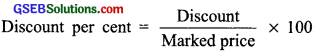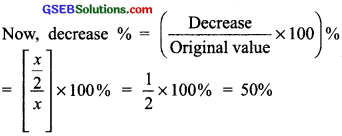# GSEB Solutions Class 8 Maths Chapter 8 Comparing Quantities InText Questions

Gujarat Board GSEB Textbook Solutions Class 8 Maths Chapter 8 Comparing Quantities InText Questions and Answers.

## Gujarat Board Textbook Solutions Class 8 Maths Chapter 8 Comparing Quantities InText Questions

Try These (Page 119)

Question 1.
In a primary school, the parents were asked about the number of hours they spend per day in helping their children to do homework. There were 90 parents who helped for $$\frac{1}{2}$$ hour to 1$$\frac{1}{2}$$ hours given in the adjoining figure; 20% helped for more than 1$$\frac{1}{2}$$ hours per day; 30% helped for $$\frac{1}{2}$$ hour to 1$$\frac{1}{2}$$ hours; 50% did not help at all. Using this, answer the following:Solution:
1. Since, 30% of total surveyed parents helped their children for hours to “$$\frac{1}{2}$$ hours to 1$$\frac{1}{2}$$ hours”. And 90 parents helped their children for “$$\frac{1}{2}$$ hours to 1$$\frac{1}{2}$$ hours”.
∴ 30% [surveyed parents] = 90
or $$\frac{30}{100}$$ × [surveyed parents] = 90
orsurveyed parents = $$\frac{90×100}{30}$$
= 3 × 100 = 300

2. Since 50% of surveyed parents did not help their children.
∴ Number of parents who did not help = 50% of surveyed parents
= 50% of 300 = $$\frac{50}{100}$$ × 300 = 150

3. Since, 20% of the surveyed parents help their children for more than 1$$\frac{1}{2}$$ hours.
i.e. 20% of surveyed parents help for more than 1$$\frac{1}{2}$$ hours.
∴ Number of parents who helped for more than 1$$\frac{1}{2}$$ hours = 20% of 300
= $$\frac{20}{100}$$ × 300 = 20 × 3 = 60
Note: ‘of’ means multiplication.

Try These (Page 121)

Question 1.
A shop gives 20% discount. What would the sale price of each of these be?
(a) A dress marked at ₹120
(b) A pair of shoes marked at ₹750
(c) A bag marked at ₹250
Solution:
(a) Marked price of the dress = ₹120
Discount rate = 20%
∴ Discount = 20% of ₹120 = ₹$$\frac{20}{100}$$ × 120
= ₹2 × 12 = ₹24
∴ Sale price of the dress = [Marked price] – [Discount]
= ₹120 – ₹24 = ₹96

(b) Marked price of the pair of shoes = ₹750
Discount rate = 20%
∴ Discount = 20% of ₹750 = ₹$$\frac{20}{100}$$ × 750
= ₹2 × 75 = ₹150
Now, sale price of the pair of shoes
= [Marked price] – [Discount]
= ₹750 – ₹150 = ₹600

(c) Marked price of the bag = ₹250
Discount rate = 20%
Discount = 20% of ₹250
= ₹$$\frac{20}{100}$$ × 250 = ₹2 × 25 = ₹50
∴ Sale price of the bag = [Marked price] – [Discount]
= ₹250 – ₹50 = ₹200Question 2.
A table marked at ₹15,000 is available for ₹14,400. Find the discount given and the discount per cent?
Solution:
Marked price of the table = ₹15000
Sale price of the table = ₹14400
∴ Discount = [Marked price] – [Sale price]
= [₹ 15000] – [₹ 14400] = ₹ 600= $$\frac{600}{15000}$$ × 100% = 4%

Question 3.
An almirah is sold at ₹5,225 after allowing a discount of 5%. Find its marked price?
Solution:
Sale price of the almirah = ₹ 5225
Discount rate = 5%
Since, Discount = 5% of marked price
∴ [Marked price] – Discount = Sale price
or [Marked price] – $$\frac{5}{100}$$ × [Marked price] = Sale price
or Marked price × $$\frac{95}{100}$$ = ₹ 5225
or Marked price = ₹ 5225 × $$\frac{100}{95}$$ = ₹ 5500

Try These (Page 123)

Question 1.
Find selling price (SP) if a profit of 5% is made on
(a) a cycle of ₹ 700 with ₹ 50 as overhead charge.
(b) a lawn mower bought at ₹ 1150 with ₹ 50 as transportation charges.
(c) a fan bought for ₹ 560 and expenses of ₹ 40 made on its repairs.
Solution:
(a) ∵ Total cost price = ₹ 700 + ₹ 50
Profit = 5% of ₹ 750
= ₹ $$\frac{5}{100}$$ × ₹ 780 = ₹ $$\frac{5×15}{2}$$
= ₹ $$\frac{75}{2}$$ = ₹ 37.50
Now, SP = CP + Profit = ₹ 750 + ₹ 37.50
= ₹ 787.50

(b) ∵ Total cost price = ₹ 1150 + ₹ 50
Profit = 5% of ₹ 1200 = $$\frac{5}{100}$$ × ₹ 1200
= ₹ 5 × 12 = ₹ 60
Now, SP = CP + Profit
= ₹ 1200 + ₹ 60
= ₹ 1260

(c) ∵ Total cost price = ₹ 560 + ₹ 40 (Overhead expenses)
= ₹ 600
Profit = 5% of ₹ 600 = $$\frac{5}{100}$$ × ₹ 600
= ₹ 5 × 6 = ₹ 30
Now, SP = CP + Profit
= ₹ 600 + ₹ 30 = ₹ 630Question 2.
A shopkeeper bought two TV sets at ₹ 10,000 each. He sold one at a profit 10% and the other at a loss of 10%. Find whether he made an overall profit or loss?
Solution:
Total CP of both TV’s = 2 × ₹ 10,000
= ₹ 20,000
For 1st TV: CP = ₹ 10,000.
Profit rate = 10%
∴ Profit = 10% of ₹ 10,000
= $$\frac{10}{100}$$ × ₹ 10,000 = ₹ 1000
∴ SP = ₹ 10,000 + ₹ 1000
= ₹ 11,000

For 2nd TV: CP = ₹ 10,000
Loss rate = 10%
∴ Loss = 10% of ₹ 10,000
= $$\frac{10}{100}$$ × ₹ 10,000 = ₹ 1000
∴ SP = CP – Loss
= ₹ 10,000 – ₹ 1000 = ₹ 9000
Now, total SP of both the TV’s
= ₹ 11,000 + ₹ 9000 = ₹ 20,000
∵ Cost price = Selling price
∴ There is no overall profit or loss.

Try These (Page 124)

Question 1.
Find the buying price of each of the following when 5% ST is added on the purchase of
(a) A towel at ₹ 50
(b) Two bars of soap at ₹ 35 each
(c) 5 kg of flour at ₹ 15 per kg
Solution:
(a) Cost of the towel = ₹ 50
∴ Sales tax on the towel = 5% of ₹ 50
= ₹ $$\frac{5}{100}$$ × 50 = ₹ 2.5
Thus, the buying price of the towel
= ₹ 50 + ₹ 2.5 = ₹ 52.5

(b) ∵ Cost of the two soap bars
= (₹ 35) × 2 = ₹ 70
∴ Sales tax = 5% of ₹ 70
= ₹ $$\frac{5}{100}$$ × 70 = ₹ 3.5
Thus, the buying price = ₹ 70 + ₹ 3.5
= ₹ 73.5

(c) ∵ Cost of 5 kg of flour = ₹ 15 × 5 = ₹ 75
∴ Sales tax = 5% of ₹ 75 = ₹ $$\frac{5}{100}$$ × 75
= ₹ $$\frac{375}{100}$$ = ₹ 3.75
Thus, the buying price = ₹ 75 + ₹ 3.75
= ₹ 78.75Question 2.
If 8% VAT is included in the prices, find the original price of
(a) A TV bought for ₹ 13,500
(b) A shampoo bottle bought for ₹ 180
Solution:
(a) Cost of TV including VAT = ₹ 13500
Rate of VAT = 8%
Let the original price = ₹ x
∴ x + 8% of x = ₹ 13,500
or x + $$\frac{8}{100}$$ x = ₹ 13,500
or x[1 + $$\frac{8}{100}$$] = ₹ 13,500
or x × $$\frac{108}{100}$$ = ₹ 13,500
or x = ₹ $$\frac{13500×100}{108}$$ = ₹ 12500
Thus, the original price = ₹ 12500.

(b) Let original price be x
∴ Price including VAT = ₹ x + 8% of x
= ₹ [1 + $$\frac{8}{100}$$]x = ₹ $$\frac{108}{100}$$x
But the original price + VAT = ₹ 180
∴ $$\frac{108}{100}$$x = ₹ 180
or x = ₹ 180 × $$\frac{100}{108}$$ = ₹ $$\frac{500}{3}$$ = ₹ 166 $$\frac{2}{3}$$
Thus, the original price = ₹ 166 $$\frac{2}{3}$$

Try These (Page 125)

Question 1.
Two times a number is a 100% increase in the number. If we take half the number, what would be the decrease in per cent?
Solution:
Let the number be x.
∴ Decrease in the number = $$\frac{1}{2}$$ of x = $$\frac{x}{2}$$Question 2.
By what percent is ₹ 2,000 less than ₹ 2,400? Is it the same as the percent by which ₹ 2,400 is more than ₹ 2,000?
Solution:
Case I
Here 2000 < 2400.
i.e., 2400 is reduced to 2000.
∴ Decrease = 2400 – 2000 = 400
Thus, decrease % = $$\frac{400}{2400}$$ × 100%
= $$\frac{50}{3}$$ % = 16 $$\frac{2}{3}$$ %

Case II
2000 is increased to 2400.
∴ Increase = 2400 – 2000 = 400
∴ Increase % = $$\frac{400}{2000}$$ × 100%
Thus, increase % = 20%
Obviously, they are not the same.

Try These (Page 126)

Question 1.
Find interest and amount to be paid on ₹ 15000 at 5% per annum after 2 years?
Solution:
Here, principal = ₹ 15000
Rate of interest = 5% per annum
Time = 2 years
∵ SI = $$\frac{P×R×T}{100}$$ ∴ SI = ₹ $$\frac{15000×5×2}{100}$$
= ₹ (150 × 5 × 2) = ₹ 1500
∵ Amount = P + SI
∴ Amount = ₹ 15000 + ₹ 1500 = ₹ 16500
Note:
I. When interest is calculated on the amount of previous year then it is called compound interest (CI).
II. Unlike simple interest, the formula for compound interest, i.e.,
A = P[1 + $$\frac{R}{100}$$]n gives us the amount directly.

Try These (Page 129)

Question 1.
Find CI on a sum of ₹ 8000 for 2 years at 5% per annum compounded annually?
Solution:
We have P = ₹ 8000, R = 5% p.a., T = 2 years
∵ A = P[1 + $$\frac{R}{100}$$]n
∴ A = ₹ 8000 [1 + $$\frac{5}{100}$$]n
= ₹ 8000 $\frac{21}{20}$2 = ₹ 8000 × $$\frac{21}{20}$$ × $$\frac{21}{20}$$
= ₹ (20 × 21 × 21) = ₹ 8820
Now, compound interest = A – P
= ₹ 8820 – ₹ 8000 = ₹ 820
= ₹ 210000 × $$\frac{19}{20}$$ × $$\frac{19}{20}$$
= ₹ (525 × 19 × 19) = ₹ 1, 89, 525
∴ The value of the car after 2 years = ₹ 1, 89, 525

Try These (Page 130)

Question 1.
Find the time period and rate for each?
1. A sum taken for 1$$\frac{1}{2}$$ years at 8% per annum is compounded half yearly.
2. A sum taken for 2 years at 4% per annum compounded half yearly.
Solution:
1. We have interest rate 8% per annum for 1$$\frac{1}{2}$$ year.
∵ It is compounded half yearly.
∴ Time period (n) = 2(1$$\frac{1}{2}$$) = 3 half years
Rate (R) = $$\frac{1}{2}$$ (8%) = 4% per half year.

2. We have interest rate 4% per annum for 2 years.
∴ Time period (n) = 2(2) = 4 half years
Rate (R) = $$\frac{1}{2}$$ (4%) = 2% per half year.

Try These (Page 131)

Question 1.
Find the amount to be paid
1. At the end of 2 years on ₹ 2,400 at 5% per annum compounded annually.
2. At the end of 1 year on ₹ 1,800 at 8% per annum compounded quarterly.
Solution:
1. We have: P = ₹ 2400, R = 5% p.a.,
T = 2 years
∵ Interest is compounded annually i.e., n = 2
∴ A = P[1 + $$\frac{R}{100}$$n
A = ₹ 2400 [1 + $$\frac{5}{100}$$2
= ₹ 2400 [$$\frac{21}{20}$$2 = ₹ 2400 × $$\frac{21}{20}$$ × $$\frac{21}{20}$$
= ₹ (6 × 21 × 21) = ₹ 2646

2. Here, interest compounded quarterly.
∴ R = 8% p.a. = $$\frac{8}{4}$$ %, i.e; 2% per quarter
T = 1 year = 4 × 1, i.e; 4 quarters or n = 4
Now A = P[1 + $$\frac{R}{100}$$n
= ₹ 1800 [1 + $$\frac{2}{100}$$4 = ₹ 1800 $$\frac{51}{50}$$4
= ₹ 1800 × $$\frac{51}{50}$$ × $$\frac{51}{50}$$ × $$\frac{51}{50}$$ × $$\frac{51}{50}$$
= $$\frac{18 \times .51 \times 51 \times 51 \times 51}{5 \times 5 \times 50 \times 50}$$
= $$\frac{121773618}{62500}$$ = ₹ 1948. 38

Try These (Page 133)

Question 1.
A machinery worth ₹ 10,500 depreciated by 5%. Find its value after one year?
Solution:
Here, P = ₹ 10,500, R = -5% p.a.,
T = 1 year, n = 1
∵ A = P[1 – $$\frac{5}{100}$$1
[∵Depreciation is there, ∴r = -5%
∴ A = ₹ 10500 [1 – $$\frac{5}{100}$$]1
= ₹10500 × $$\frac{19}{20}$$ = ₹ 525 × 19 = ₹ 9975
Thus, machinery value after 1 year = ₹ 9975
Note: For depreciation, we use the formula as
A = P[1 – $$\frac{R}{100}$$]nQuestion 2.
Find the population of a city after 2 years, which is at present 12 lakh, if the rate of increase is 4%?
Solution:
Present population, P = 12 lakh
Rate of increase, R = 4% p.a.
Time, T = 2 years ∴ n = 2
∴ Population after 2 years = P[1 + $$\frac{R}{100}$$n
= 12 lakh[1 + $$\frac{4}{100}$$2 = 12 Lakh $$\frac{26}{25}$$2
= 12,00,000 × $$\frac{26}{25}$$ × $$\frac{26}{25}$$ = 1920 × 26 × 26
= 12,97,920
Thus, the population of the town will be 12,97,920 after 2 years.